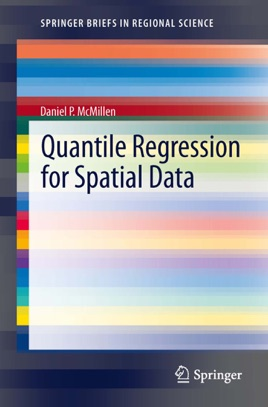• 44,99 €

## Descrizione dell’editore

Quantile regression analysis differs from more conventional regression models in its emphasis on distributions. Whereas standard regression procedures show how the expected value of the dependent variable responds to a change in an explanatory variable, quantile regressions imply predicted changes for the entire distribution of the dependent variable.  Despite its advantages, quantile regression is still not commonly used in the analysis of spatial data. The objective of this book is to make quantile regression procedures more accessible for researchers working with spatial data sets. The emphasis is on interpretation of quantile regression results. A series of examples using both simulated and actual data sets shows how readily seemingly complex quantile regression results can be interpreted with sets of well-constructed graphs.  Both parametric and nonparametric versions of spatial models are considered in detail.